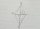# Rhombus

Calculate the perimeter and area of ​​a rhombus whose diagonals are 38 cm and 55 cm long.

Result

p =  133.7 cm
A =  1045 cm2

#### Solution:Leave us a comment of example and its solution (i.e. if it is still somewhat unclear...):Be the first to comment!#### To solve this example are needed these knowledge from mathematics:

Pythagorean theorem is the base for the right triangle calculator.

## Next similar examples:

1. Triangle SASCalculate the area and perimeter of the triangle, if the two sides are 51 cm and 110 cm long and angle them clamped is 130 °.
2. Ratio of sidesCalculate the area of a circle that has the same circumference as the circumference of the rectangle inscribed with a circle with a radius of r 9 cm so that its sides are in ratio 2 to 7.
3. Right ΔA right triangle has the length of one leg 28 cm and length of the hypotenuse 53 cm. Calculate the height of the triangle.
4. Axial sectionAxial section of the cone is an equilateral triangle with area 208 dm2. Calculate the volume of the cone.
5. SquarePoints A[-5,-6] and B[7,-1] are adjacent vertices of the square ABCD. Calculate the area of the square ABCD.
6. CubesOne cube is inscribed sphere and the other one described. Calculate difference of volumes of cubes, if the difference of surfaces in 257 mm2.
7. Cone A2VSurface of cone in the plane is a circular arc with central angle of 126° and area 415 cm2. Calculate the volume of a cone.
8. RiverCalculate how many promiles river Dunaj average falls, if on section long 957 km flowing water from 1454 m AMSL to 101 m AMSL.
9. GardenArea of a square garden is 6/4 of triangle garden with sides 56 m, 35 m, and 35 m. How many meters of fencing need to fence a square garden?
10. Tetrahedral pyramidWhat is the surface of a regular tetrahedral (four-sided) pyramid if the base edge a=7 and height v=6?
11. A kiteABCD is a kite. Angle OBC = 20° and angle OCD = 35°. O is the intersection of diagonals. Find angle ABC, angle ADC and angle BAD.
12. RectangleThe rectangle is 11 cm long and 45 cm wide. Determine the radius of the circle circumscribing rectangle.
13. Rectangular trapezoidIn a rectangular trapezoid ABCD with right angles at vertices A and D with sides a = 12cm, b = 13cm, c = 7cm. Find the angles beta and gamma and height v.
14. Circle chordWhat is the length d of the chord circle of diameter 36 m, if the distance from the center circle is 16 m?
15. MovementFrom the crossing of two perpendicular roads started two cyclists (each at different road). One runs at average speed 28 km/h, the second at average speed 24 km/h. Determine the distance between them after 45 minutes cycling.
16. Is right?Is triangle with sides 51, 56 and 77 right triangle?
17. Short cutImagine that you are going to the friend. That path has a length 330 meters. Then turn left and go another 2000 meters and you are at a friend's. The question is how much the journey will be shorter if you go direct across the field?